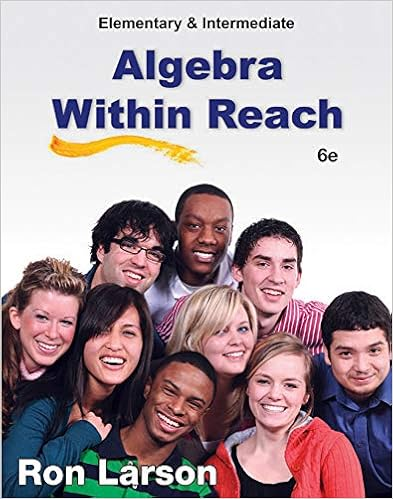# Answer a standard cost per urn 4800 800 600 per urn

• Test Prep
• 68
• 96% (665) 640 out of 665 people found this document helpful

This preview shows page 51 - 54 out of 68 pages.

##### We have textbook solutions for you!
The document you are viewing contains questions related to this textbook.The document you are viewing contains questions related to this textbook.
Chapter 3 / Exercise 81
Elementary and Intermediate Algebra: Algebra Within Reach
LarsonExpert Verified
Answer: a.Standard cost per urn= \$4,800 / 800= \$6.00 per urn Standard number of pounds per urn= \$6.00 / \$4.80= 1.25 pound per urn b.Materials price variance= Total variance - efficiency variance= (\$4,480 − \$4,800) − \$96 unfavorable= \$416 favorable c.Total standard labor cost of actual hours= ((800/2) × \$19.2) − \$288 favorable= \$7,392Actual hours= \$7,392/19.2 = 385 hoursTotal actual costs= 385 × \$19.60 = \$7,546 d.Labor price variance= \$7,546 − \$7,392= \$154 unfavorable Diff: 3Objective: 5AACSB: Application of knowledge
##### We have textbook solutions for you!
The document you are viewing contains questions related to this textbook.The document you are viewing contains questions related to this textbook.
Chapter 3 / Exercise 81
Elementary and Intermediate Algebra: Algebra Within Reach
LarsonExpert Verified
44) The following data for the Panoid Garden Supplies Company pertains to the production of 2,000 garden spades during March. The spade consists of a wooden handle and a metal forged tool that comes in contact with the ground.Direct Materials (all materials purchased were used): Standard cost: \$1.00 per handle and \$3.00 per metal tool.Total actual cost: \$9,000.Materials flexible-budget efficiency variance was \$500 unfavorable. Direct Manufacturing Labor: Standard cost is 5 garden spades per hour at \$20.00 per hour.Actual cost per hour was \$21.00.Labor efficiency variance was \$500 favorable. Required: a.What is the standard direct material amount per garden spade?b.What is the standard cost allowed for all units produced?c.What is the total direct materials flexible-budget variance?d.What is the direct material flexible-budget price variance?e.What is the total actual cost of direct manufacturing labor?f.What is the labor price variance for direct manufacturing labor? Answer: a.Standard cost per garden spade= \$1.00 (handle) + \$3.00 (tool)= \$4.00 per garden spadeb.Standard cost allowed for all units= 2,000 × \$4.00= \$8,000 per garden spadec.Total materials variance = \$8,000 − \$9,000= \$1,000 unfavorable= \$1,000 unfavorabled.Materials price variance= Total variance - efficiency variance= (\$9,000 − \$8,000) − \$500 unfavorable= \$500 unfavorable e.Total standard labor cost of actual hours= ((2,000/5) × \$20) − \$500 favorable= \$7,500Actual hours= \$7,500/20 = 375 hoursTotal actual costs= 375 × \$21 = \$7,875 f.Labor price variance= \$7,500 − \$7,875= \$375 unfavorable Diff: 3Objective: 5AACSB: Application of knowledge45) The following data for the telephone company pertain to the production of 450 rolls of telephone wire during June. Selected items are omitted because the costing records were lost in a windstorm. Direct Materials (All materials purchased were used.) Standard cost per roll: a pounds at \$4.00 per pound.Total actual cost: b pounds costing \$9,600. Standard cost allowed for units produced was \$9,000.
Materials price variance: c .Materials efficiency variance was \$80 unfavorable. Direct Manufacturing Labor Standard cost is 3 hours per roll at \$8.00 per hour.Actual cost per hour was \$8.25.Total actual cost: d .Labor price variance: e .Labor efficiency variance was \$400 unfavorable. Required:Compute the missing elements in the report represented by the lettered items. Answer: a.Standard cost per roll= \$9,000/450 = \$20.00 Standard number of pounds per roll= \$20/\$4 = 5 pounds per roll b.Actual pounds= (\$9,000 + \$80)/\$4= 2,270 pounds c.Materials price variance= \$9,600 - (\$9,000 + \$80)= \$520 unfavorable d.Total standard labor cost of actual hours= (450 × 3 × \$8) + \$400 = \$11,200Actual hours= \$11,200/\$8 = 1,400 Total actual cost= 1,400 × \$8.25= \$11,550 e.Labor price variance= \$11,550 - \$11,200= \$350 unfavorable Diff: 3Objective: 5AACSB: Application of knowledge
•••